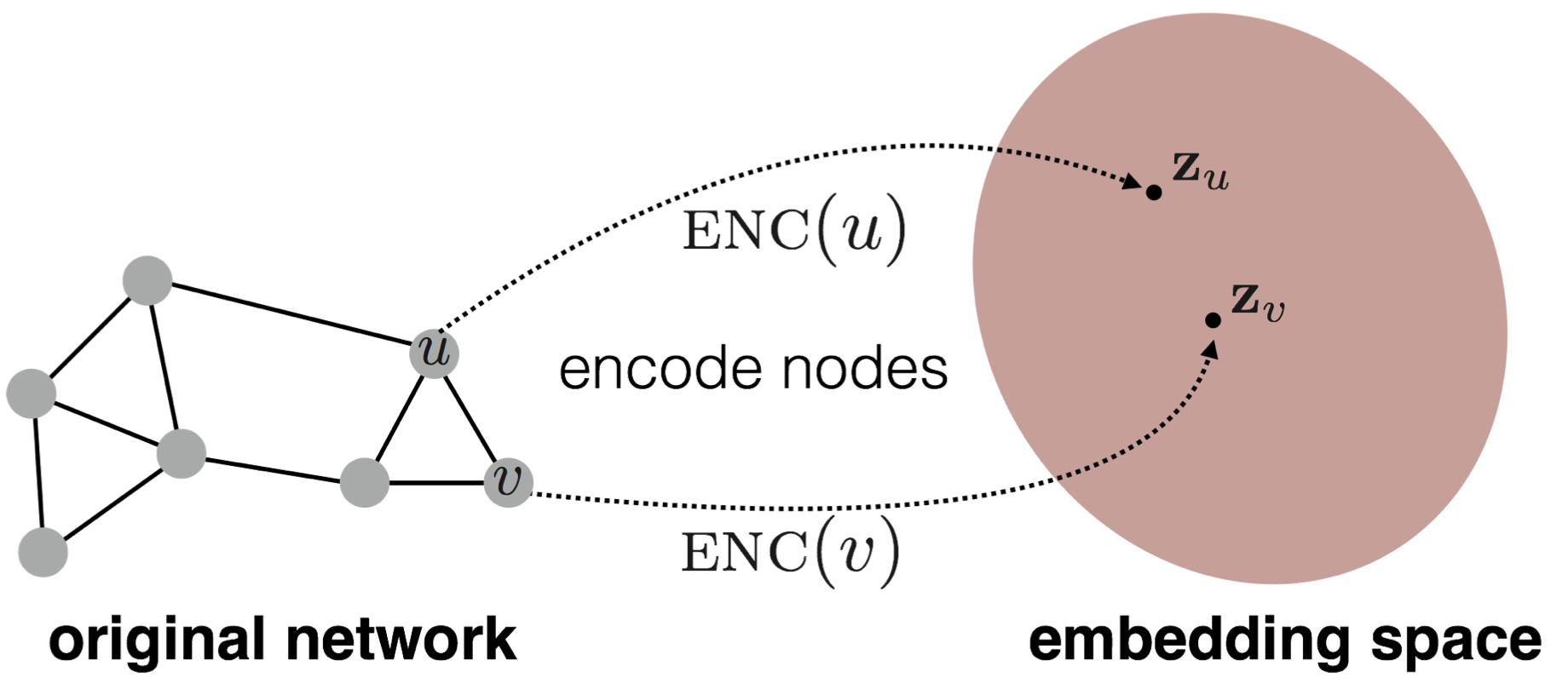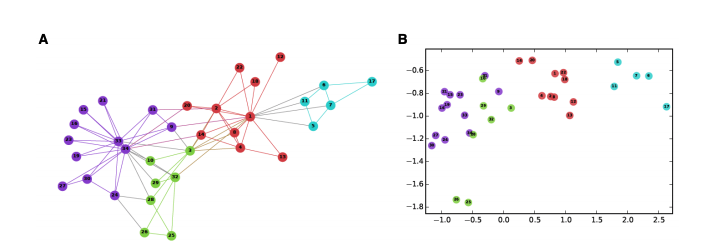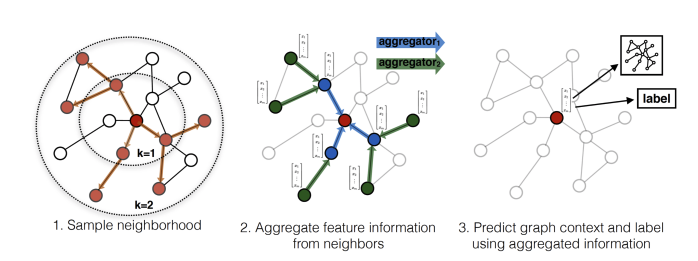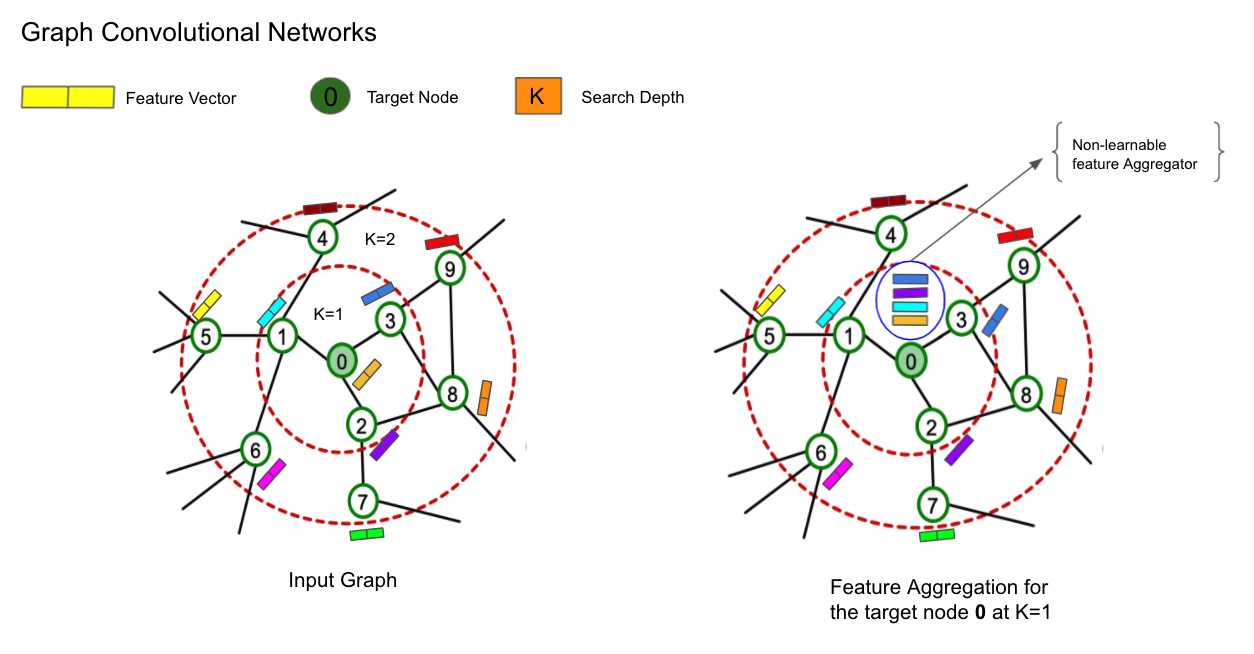Estimated reading time: 15 minute

This blog post provides a comprehensive study on the theoretical and practical understanding of GraphSage, this notebook will cover:

• What is GraphSage
• Neighbourhood Sampling
• Getting Hands-on Experience with GraphSage and PyTorch Geometric Library
• Open-Graph-Benchmark’s Amazon Product Recommendation Dataset
• Creating and Saving a model
• Generating Graph Embeddings Visualizations and Observations

## Important Note!!¶

If you are running this notebook on Google Colab, please make sure to enable hardware acceleration using either a GPU or a TPU. If it is run with CPU-only enabled, generating the word embeddings will take an incredibly long time! Hardware acceleration can be enabled by navigating to `Runtime` -> `Change Runtime`. This will present you with a popup, where you can select an appropriate `Hardware Accelerator`.

# A Comprehensive Case-Study of GraphSage Algorithm with Hands-on-Experience using PyTorchGeometric Library and Open-Graph-Benchmark's Amazon Product Recommendation Dataset¶

This notebook/blogpost provides a comprehensive study on theoretical and practical understanding of GraphSage which is an inductive representation learning algorithm. For a practical application we are going to use the popular PyTorch Geometric library and Open-Graph-Benchmark dataset.

We use obgn-products dataset which is an undirected and unweighted graph, representing an Amazon product co-purchasing network to predict shopping preferences. Nodes represent products sold in Amazon, and edges between two products indicate that the products are purchased together. The goal is to predict the category of a product in a multi-class classification setup, where the 47 top-level categories are used for target labels making it a Node Classification Task.

# What is Graph Representation Learning?¶

Once the graph is created after incorporating meaningful relationships (edges) between all the entities (nodes) of the graph. The next question comes into mind is finding a way to integrate the information about graph structure (for e.g. information about the node's global position in the graph or its local neighbourhood structure) into a machine learning model. One way to extract structural information from the graph is to compute its graph statistics using node degrees, clustering coefficients, kernel functions or hand-engineered features to estimate local neighbourhood structures. However, with these methods we can not perform an end-to-end learning i.e features cannot be learned with the help of loss function during the training process.

To tackle the above problem, representation learning approaches have been adopted to encode the structural information about the graphs into the euclidean space (vector/embedding space). The key idea behind the graph representation learning is to learn a mapping function that embeds nodes, or entire (sub)graphs (from non-euclidean), as points in low-dimensional vector space (to embedding space). The aim is to optimize this mapping so that nodes which are nearby in the original network should also remain close to each other in the embedding space (vector space), while shoving unconnected nodes apart. Therefore by doing this, we can preserve the geometric relationships of the original network inside the embedding space by learning a mapping function. Below diagram (reference: stanford-cs224w) depicts the mapping process, encoder enc maps node u and v to low-dimensional vector zu and zv :Lets understand this more intutively with an interesting example from the graph structure of the Zachary Karate Club social network. In this graph, the nodes represent the persons and there exists an edge between the two persons if they are friends. The coloring in the graph represents different communities. In the below figure A) represents the Zachary Karate Club social network and B) illustrates the 2D visualization of node embeddings created from the Karate graph using a DeepWalk method. If you analyze both the diagrams you will find that the mapping of nodes from a graph structure (non-euclidean or irregular domain) to an embedding space (figure B) is done in such a manner that the distances between nodes in the embedding space mirrors closeness in the original graph (preserving the structure of the node’s neighborhood). For e.g the community of the people marked as violet and green shares close proximity in the karate graph as compared to the communities violet and seagreen which are far away from each other. When the DeepWalk method is applied on the karate graph (in order to learn the node embeddings) we can observe the same proximity behaviour when the learned node embeddings are visualized on 2D space.

## We can use these learned node embeddings for various machine learning downstream tasks:¶

1) It can be used as a feature inputs for downstream ML tasks (eg. community detection via node classification or link prediction)

2) We could construct a KNN/Cosine similarity graph from embeddings. The graph could be used to make recommendations (e.g product recommedation)

3) Visual exploration of data by reducing them to 2 or 3 dimensions using U-Map, t-SNE algorithms (eg. performing clustering).

4) Dataset Comparisons

5) Transfer Learning# GraphSage Motivation!!¶

In this blogpost/notebook, we will cover a GraphSage (Sample and Aggregate) algorithm which is an inductive (it can generalize to unseen nodes) deep learning method developed by Hamilton, Ying, and Leskovec (2017) for graphs used to generate low-dimensional vector representations for nodes. This is in contrast with the previous graph machine learning methods like Graph Convolutional Networks or DeepWalk which are inherently transductive i.e they can only generate embeddings for the nodes present in the fixed graph during the training.
This implies that, if in future the graph evolves and new nodes (unseen during the training) make their way into the graph then we need to retrain the whole graph in order to compute the embeddings for the new node. This limitation makes the transductive approaches inefficient to get applied on the ever evolving graphs (like social networks, protein-protein networks, etc) because of their inability to generalize on unseen nodes. The other main limitation of transductive approaches is that they cannot leverage the node features e.g text attributes, node profile information, node degrees, etc.
On the other hand, the GraphSage algorithm exploits both the rich node features and the topological structure of each node’s neighborhood simultaneously to efficiently generate representations for new nodes without retraining.

# Working Principles of GraphSage¶The working process of GraphSage is mainly divided into two steps, first performing neighborhood sampling of an input graph and the second one is learning aggregation functions at each search depth. We will discuss each of these steps in detail starting with a little motivation of what was the need to perform the sampling of nodes neighborhood. Afterwards, we will discuss the importance of learning aggregator functions which basically had helped GraphSage algorithm to achieve its property of inductiveness.

## What is the importance of Neighborhood Sampling?¶

Lets understand this from the perspective of Graph Convolutional Network diagram (GCNs) described below. GCNs is an algorithm which can leverage both the graph topological information (i.e. node's neighborhood) and node features and then distill this information in order to generate node representations or dense vector embeddings. The below diagram represents the working process of GCNs intuitively. On the left hand side we have a sample input graph where its nodes are represented by their corresponding feature vectors (for e.g. node degree or text embeddings, etc). We start with defining a search depth (K) which informs the algorithm upto what depth it should gather the information from the neighborhood of a target node. Here, K is a hyperparameter and it also depicts the number of layers used in the GCNs.

At K=0, GCNs initializes all the node embeddings to their original feature vector. Now, let's say we want to compute the embeddings for the target node 0 at layer K=1, then we aggregate (it is permutation invariant function to its neighbors) all the feature vectors of nodes (including itself) which are at 1-hop distance from the node 0 (at this timestep or layer we are aggregating the original feature representations of nodes which are at K=0). For the target node 0, GCNs uses a mean aggregator to compute the mean of the neighborhood node features along with its own features (self-loop). After K=1, the target node 0 now knows about the information about its immediate neighborhood; this process is shown below in the GCNs image (r.h.s). We repeat this process for all the nodes in the graph (i.e. for every node, we aggregate over the 1-hop neighborhood) in order to find the new representations for each node at each layer.

Note: As the search depth increases, the reach of the target node in terms of aggregating features from its local neighborhood also increases. For e.g. at K=1 the target node knows the information about its local neighborhood which are 1-hop distance, at K=2 the target node knows the information about its local neighborhood which are at 1-hop distance and the neighbors of the nodes of 1-hop distance i.e upto 2-hop distance.## Issues with GCN approach¶

As we have discussed above, that GCNs compute node representations using neighborhood aggregation. For the training purposes, we can represent the k-hop neighborhood of a target node as a computational graph and send these computational graphs in a mini-batch fashion in order to learn the weights of the network (i.e. applying stochastic gradient descent). The below diagram illustrates a computational graph for the target node 0 upto 2-hop neighborhood. Now, the problem with this is that:

1) Computationally Expensive: Since for each node, we need to generate the complete K-hop neighborhood computational graph and then need to aggregate plenty of information from its surroundings. As we go deeper into the neighborhood (large K) computation graph becomes exponentially large. This could lead to a problem while fitting these big computational graphs inside GPU memory.

2) The curse of Hub nodes or Celebrity nodes: Hub nodes are those nodes which are very high degree nodes in the graph for eg. a very popular celebrity having millions of connections. If that is the case then we need to aggregate the information from millions of nodes in order to compute the embeddings for the hub node. Therefore, the generated computational graph for the hub node is very huge. This problem is illustrated below diagramatically (r.h.s).